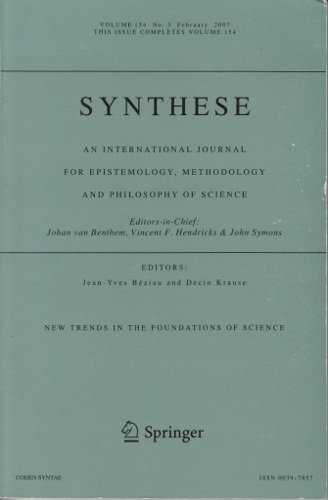## On the Epistemological Analysis of Modeling and Computational Error in the Mathematical SciencesThis paper developed in collaboration with Rob Corless examines aspects of the significance of backward error analysis for our understanding of the rationality of scientific practice.

Title:
On the Epistemological Analysis of Modeling and Computational Error in the Mathematical Sciences
Authors:
Nicolas Fillion and Robert M. Corless
Journal:
Synthese

Abstract: Interest in the computational aspects of modeling has been steadily growing in philosophy of science. This paper aims to advance the discussion by articulating the way in which modeling and computational errors are related and by explaining the signi cance of error management strategies for the rational reconstruction of scienti c practice. To this end, I rst characterize the role and nature of modeling error in relation to a recipe for model construction known as Euler's recipe. I then describe a general model that allows us to assess the quality of numerical solutions in terms of measures of computational errors that are completely interpretable in terms of modeling error. Finally, I emphasize that this type of error analysis involves forms of perturbation analysis that go beyond the basic model-theorical and statistical/probabilistic tools typically used to characterize the scienti c method; this demands that we revise and complement our reconstructive toolbox in a way that can a ect our normative image of science.

Cite as (APA format):
Fillion, N. & Corless, R.M. (2014). On the Epistemological Analysis of Modeling and Computational Error in the Mathematical Sciences, Synthese, 191: 1451-1467.

BibTeX Entry:
@article{Fillion2014,
title={On the Epistemological Analysis of Modeling and Computational Error in the Mathematical Sciences},
author={Fillion, Nicolas and Corless, Robert~M.},
journal={Synthese},
volume={191},
pages={1451--1467},
year={2014},
publisher={Springer}
}

tags: